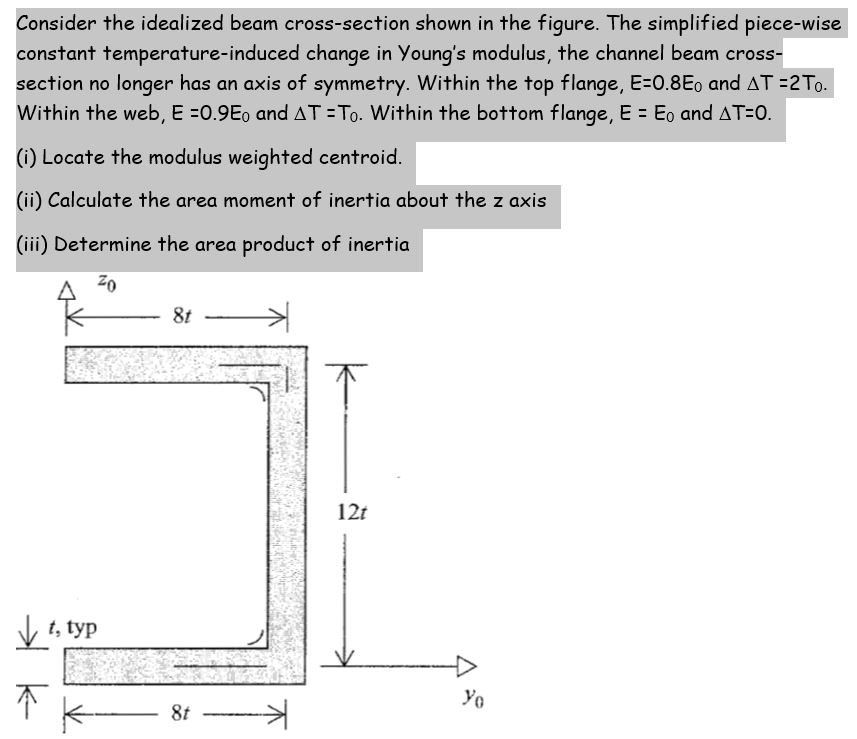# Consider the idealized beam cross-section shown in the figure. The simplified piece-wise constant temperature-induced change in...

###### Question:Consider the idealized beam cross-section shown in the figure. The simplified piece-wise constant temperature-induced change in Young's modulus, the channel beam cross- section no longer has an axis of symmetry. Within the top flange, E=0.8Eo and AT =2 To. Within the web, E =0.9Eo and AT = To. Within the bottom flange, E = Eo and AT-O. (i) Locate the modulus weighted centroid. (ii) Calculate the area moment of inertia about the z axis (iii) Determine the area product of inertia 20 8t 121 t, typ yo 8t *

#### Similar Solved Questions

##### 2. Find the equation of a line which is perpendicular to the line 2x-y=4 and include...
2. Find the equation of a line which is perpendicular to the line 2x-y=4 and include P(3,4) 3. Find the equation of a cirle with the radius 3 and the center (1,-1) 2.5 4. Find the radius and the center of the following cicle equation 05 x² + y² - 2x + 4y - 4 = 0 1. GÖ...
##### I need help with creating the following: Write down a research question. Make sure that your que...
I need help with creating the following: Write down a research question. Make sure that your question involves two variables. What variable names are involved with your research question? You can name your variables. Invent 10 data values to describe each of your variables and include the data here...
##### Risk-adjusted rates of return using CAPM Centennial Catering, Inc., is considering two mutually exclusive investments. The...
Risk-adjusted rates of return using CAPM Centennial Catering, Inc., is considering two mutually exclusive investments. The company wishes to use a CAPM-type risk-adjusted discount rate (RADR) in its analysis. Centennial's managers believe that the appropriate market rate of return is 12.3%, and ...
##### How do you solve 4| w + 7| + 6\leq 14?
How do you solve 4| w + 7| + 6\leq 14?...